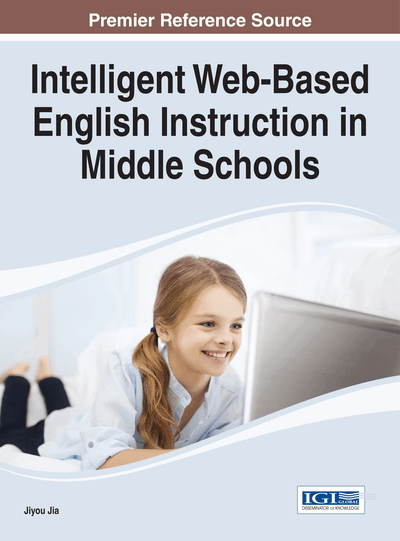# The Analysis of Examination Scores

DOI: 10.4018/978-1-4666-6607-8.ch005
Available
\$37.50
No Current Special Offers

## Abstract

This chapter analyzes the scores of experiment and control students in four middle schools in regular and vocabulary tests from September 2010 to July 2012. Descriptive statistics are calculated and shown in tables and line charts, including means, standard deviations, T-tests, and effect sizes comparing the means of experiment and control class in the same exam. If both vocabulary pretest and posttest with the same test content were conducted, the T-test comparing the means of pretest and posttest and the effect size are also calculated. The statistical findings demonstrate that the blended learning with CSIEC system has more positive effect on students' learning achievement in regular examinations and especially in vocabulary acquisition than the traditional approach. The quasi-experiments verified the positive effect's reliability and validity regarding the equivalence of the treatment and control class, the participants' ages/grades, the teachers' experiences, the learning materials, and the schools' locations and teaching qualities.
Chapter Preview
Top

## Introduction

To measure the learning performance of the students, this chapter focuses on test scores for two practical reasons. At first, student test scores are a commonly reported measure of learning in educational studies, and an indicator used for a comparative analysis. Secondly, as the student testing is traditionally important in China and other countries, the effect of a new technology on student test scores is an increasingly relevant criterion for public policy-makers to decide between alternative educational interventions.

During the two years’ empirical research from September 2010 to July 2012, the research team attempted to collect all the papers and grades of regular and proctored examinations from the four high schools, including final term exams as a must, midterm exams and monthly exams if possible. Because the content and difficulty of the regular exams in different time are different from each other, it is more reasonable to compare the mean difference of student scores in the same examination between the treatment class and control class than to compare the longitudinal variance of the score mean of each class. Furthermore, independent sample T-test (Hazewinkel, 2001; Zhang, 2009) was calculated to examine the statistical significance of the mean difference between the treatment and control class. The effect of the blended learning with the CSIEC system can be inferred based on the analysis of mean difference variance in pretest and posttest. The pretest was usually defined as the last exam preceding the experiment, for example the final exam in the previous term, or the entrance exam for the first grade. If it was difficult to obtain all the students’ scores in last term, the first exam in the experiment term was adopted as the pretest. The posttest was usually the last or final exam in the experiment school term or school year. The usage of regular exams in the schools instead of specifically designed exams can avoid the unnecessary disturbance to the schools’ teaching schedule and order.

In addition to regular exams, the research team designed an online quiz to measure the students’ vocabulary ability required in the experiment term, and called it vocabulary test. If this test could be scheduled at the beginning of the experiment term, it was the pretest for the vocabulary mastery. If it was scheduled at the end of the experiment, it was the posttest for the vocabulary mastery. If the school could schedule the online vocabulary posttest and/or pretest for both classes, the research team also compared the mean difference between the two classes in the same posttest or pretest and the difference’s statistical significance using independent sample T-test. But if the school could not schedule the online vocabulary test for the control class, the research team just compared the historical variance of the treatment class from pretest to posttest and used paired sample T-test to calculate the difference’s statistical significance, because the content of the posttest was the same as that of the pretest.

However, t-test only measures the likelihood that any significant effect at all exists, but not the magnitude or robustness of treatment effects. Therefore, besides T-test, effect size of the blended learning with the CSIEC system was also calculated. “Effect size is a simple way of quantifying the difference between two groups that has many advantages over the use of tests of statistical significance alone.”(Coe, 2002) According to Wikipedia (http://en.wikipedia.org/wiki/Effect_size), “an effect size is a measure of the strength of a phenomenon (for example, the change in an outcome after experimental intervention). An effect size calculated from data is a descriptive statistic that conveys the estimated magnitude of a relationship without making any statement about whether the apparent relationship in the data reflects a true relationship in the population.” To estimate effect size, Cohen’s d is used, and is defined as the following (Cohen, 1988; Cohen, 1992; Aaron, Kromrey, & Ferron, 1998).

where Mean1is the mean of the exam scores of the students in experiment class, Mean2 is mean of the exam scores of the students in control class. The standard deviation can be calculated with the following formula, where n1, n2 is the sample size of the experiment and control class, respectively; and SD1, SD2 is the standard deviation of the experiment and control class, respectively.

## Complete Chapter List

Search this Book:
Reset